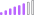cancel
Showing results for
Did you mean:Post Patron

## Calculate Percentage

Hello,

I want to calculate and find what is the percentage of the Paperless Bills among the Non Paperless Bills

I have a 2 measures that counts the paperless and non paperless. What can be my formula?

Thank you

1 ACCEPTED SOLUTIONSuper User

you can try by creating a measure and format it as Percent.

``````_Percent =

FORMAT(
DIVIDE ([counts of paperless],[count of non paperless],BLANK()) ,"Percent")``````

Proud to be a Super User!Super User

you can try by creating a measure and format it as Percent.

``````_Percent =

FORMAT(
DIVIDE ([counts of paperless],[count of non paperless],BLANK()) ,"Percent")``````

Proud to be a Super User!